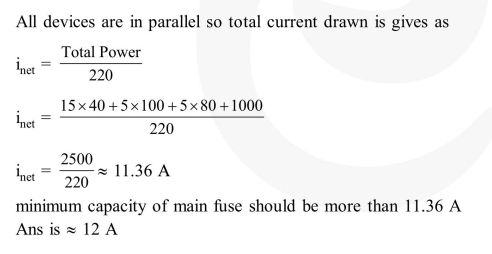# In a large building, there are 15 bulbs of

Question:

In a large building, there are 15 bulbs of $40 \mathrm{~W}, 5$ bulbs of $100 \mathrm{~W}, 5$ fans of $80 \mathrm{~W}$ and 1 heater of $1 \mathrm{~kW}$. The voltage of the electric mains is $220 \mathrm{~V}$. The minimum capacity of the main fuse of the building will be :

1. $12 \mathrm{~A}$

2.  $14 \mathrm{~A}$

3. $8 \mathrm{~A}$

4.  $10 \mathrm{~A}$

Correct Option: 1

Solution: